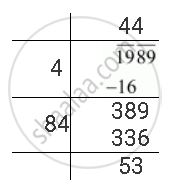Share

# Find the Least Number Which Must Be Subtracted from the Following Number So as to Get a Perfect Square. Also Find the Square Root of the Perfect Square So Obtained 1989 - CBSE Class 8 - Mathematics

ConceptSquare Roots - Finding Square Root by Division Method

#### Question

Find the least number which must be subtracted from the following number so as to get a perfect square. Also find the square root of the perfect square so obtained

1989

#### Solution

The square root of 1989 can be calculated by long division method as follows.The remainder is 53. It represents that the square of 44 is less than 1989 by 53. Therefore, a perfect square will be obtained by subtracting 53 from the given number 1989.

Therefore, required perfect square = 1989 − 53 = 1936

And sqrt(1936) = 44

Is there an error in this question or solution?

#### APPEARS IN

NCERT Solution for Mathematics Textbook for Class 8 (2018 to Current)
Chapter 6: Squares and Square Roots
Ex. 6.40 | Q: 4.2 | Page no. 108
Solution Find the Least Number Which Must Be Subtracted from the Following Number So as to Get a Perfect Square. Also Find the Square Root of the Perfect Square So Obtained 1989 Concept: Square Roots - Finding Square Root by Division Method.
S